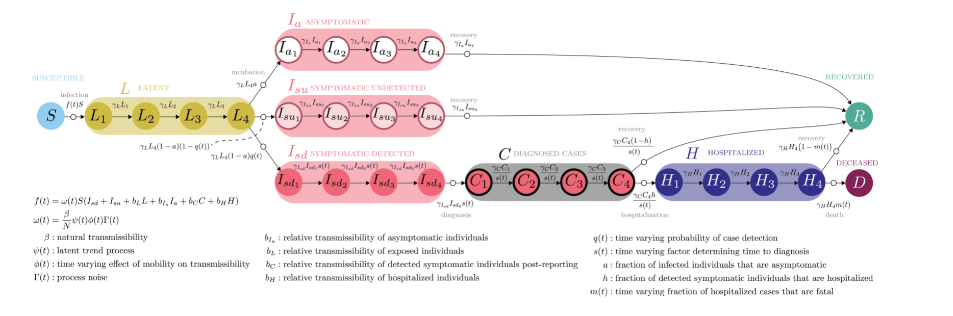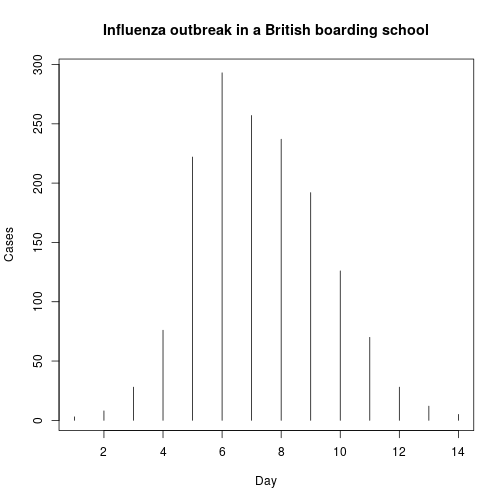# Estimation: Informing models with data

### Estimation and inference

Operationalizing models requires calibrating them to specific uses

• Calibration can be ad hoc or involve estimation of parameters from data (or a combination)
• When statistical techniques are used for estimation, then one can conduct inference (testing of hypotheses, theory confirmation)• Measuring “aggregate parameters” (e.g. $$R_0$$)
• Estimation of parameters ($$\beta$$, $$\gamma$$, etc.)
• Estimation of unknown state variables (e.g. $$X(0)$$, $$Y(0)$$, $$Z(0)$$, asymptomatic cases)

### Some approaches to measuring R0

1. Final outbreak size estimator
2. Mean age of infection
3. Epidemic takeoff
4. Doubling time

### Final outbreak size estimator

Recall from presentation on deterministic models the final size relation $1-R(\infty)-e^{-R(\infty)R_0} = 0$

This can be rearranged to give a formula for $$R_0$$ in terms of observables

$R_0 = -\frac{\log(1-R(\infty))}{R(\infty)}$

This formula is valid even when numerous assumptions underlying the simple SIR model are relaxed.

Ma, J. & D. Earn. 2006. Generality of the final size formula for an epidemic of a newly invading infectious disease. Bulletin of Mathematical Biology 68:679-702.

### Worked example$$N=764$$

$$X(0) = 763$$

$$Z(\infty) = 512$$

$$R(\infty) = Z(\infty)/X(0) \approx 0.671 \implies R_0 \approx 1.65$$

### Final outbreak size estimator

A related estimator based on cases ($$C$$):

$R_0 = \frac{N-1}{C} \sum_{i=X_f+1}^{X_0} \frac{1}{i}$

with standard error

$SE = \frac{N-1}{C} \sqrt{ \sum_{i=X_f+1}^{X_0} \frac{1}{i^2} + \frac{CR_0^2}{(N-1)^2}}$

Becker, N. 1989. Analysis of Infectious Disease Data. London: Chapman & Hall.